﻿ New Fully-Uncoupled Current-Controlled Sinusoidal Oscillator Employing Grounded Capacitors

New Fully-Uncoupled Current-Controlled Sinusoidal Oscillator Employing Grounded Capacitors

D. R. Bhaskar, D. Prasad, R. Senani, M. K. Jain, V. K. Singh, D. K. Srivastava

New Fully-Uncoupled Current-Controlled Sinusoidal Oscillator Employing Grounded Capacitors

D. R. Bhaskar1, D. Prasad1, R. Senani2,, M. K. Jain3, V. K. Singh3, D. K. Srivastava3

1Department of Electronics and Communication Engineering, Faculty of Engineering and Technology, Jamia Millia Islamia, New Delhi, India

2Division of Electronics and Communication Engineering, Netaji Subhas Institute of Technology, Sector-3, Dwarka, New Delhi, India

3Department of Electronics Engineering, Institute of Engineering and Technology, Lucknow, India

Abstract

A new electronically-controllable fully-uncoupled sinusoidal oscillator, employing four second generation current-controlled conveyors (CCCIIs) and two grounded capacitors, is presented. The oscillation frequency and condition of oscillation are totally uncoupled and electronically adjustable. The circuit has low sensitivities and a high frequency stability factor. SPICE simulation results confirm the validity of the proposed oscillators.

• D. R. Bhaskar, D. Prasad, R. Senani, M. K. Jain, V. K. Singh, D. K. Srivastava. New Fully-Uncoupled Current-Controlled Sinusoidal Oscillator Employing Grounded Capacitors. American Journal of Electrical and Electronic Engineering. Vol. 4, No. 3, 2016, pp 81-84. http://pubs.sciepub.com/ajeee/4/3/2
• Bhaskar, D. R., et al. "New Fully-Uncoupled Current-Controlled Sinusoidal Oscillator Employing Grounded Capacitors." American Journal of Electrical and Electronic Engineering 4.3 (2016): 81-84.
• Bhaskar, D. R. , Prasad, D. , Senani, R. , Jain, M. K. , Singh, V. K. , & Srivastava, D. K. (2016). New Fully-Uncoupled Current-Controlled Sinusoidal Oscillator Employing Grounded Capacitors. American Journal of Electrical and Electronic Engineering, 4(3), 81-84.
• Bhaskar, D. R., D. Prasad, R. Senani, M. K. Jain, V. K. Singh, and D. K. Srivastava. "New Fully-Uncoupled Current-Controlled Sinusoidal Oscillator Employing Grounded Capacitors." American Journal of Electrical and Electronic Engineering 4, no. 3 (2016): 81-84.

 Import into BibTeX Import into EndNote Import into RefMan Import into RefWorks

12
Prev Next

1. Introduction

Fabre, Saaid, Wiest, and Boucheron introduced ,  the second generation current controlled conveyor (CCCII) as an attractive building block for realizing electronically controllable analog signal processing circuits. Since a CCCII is based upon a four transistor based mixed translinear cell (MTC), the intrinsic X-port resistance (Rx) is electronically controllable through an external bias current (IB) with Rx being inversely proportional to IB. It is this characteristic which makes CCCII a suitable building block for electronically controllable functional circuits. Even in a CMOS realization using CMOS MTC, the tunability of Rx is available although Rx is now inversely proportional to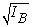. For a comprehensive account of numerous applications of CCCII such as in the realization of grounded and floating current controlled positive or negative resistances, current controlled voltage-mode/current-mode (VM/CM) amplifiers, active only summing/difference amplifiers, instrumentation amplifiers, electronically-controllable grounded/floating inductors/transformers, electronically controllable multifunction VM/CM biquads, PID controller, Precision rectifiers, CM multiplier/divider, squaring/square rooting circuits, ASK/FSK/PSK/QAM wave generator circuits, see [1-10] and the references cited therein. References [11, 12] have also elaborated upon the various current controllable sinusoidal oscillators using two to three CCCIIs.

From a survey of the existing literature on CCCIIs [1-10], it has been found that any topology for realizing fully-uncoupled oscillator capable of providing independent control of condition of oscillation (CO) and independent linear control of frequency of oscillation (FO) has not been proposed in the open literature so far. The purpose of this paper is, therefore, to present a new CCCII-based oscillator configuration which fills this void.

2. New Fully-uncoupled Current-controlled Sinusoidal Oscillator

The symbolic notation of second generation current controlled conveyor (CCCII) is shown in Fig.1. The port relationships of CCCII± can be described by the following matrix equation: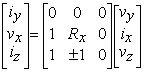(1)Download asVeiw figureFigures index
Figure 1. Symbolic notation of CCCII

In the above equation (1), Rx is the intrinsic (parasitic) resistance of CCCII which is controllable through the bias current. The positive and negative signs of the z-port current iz denote the positive (CCCII+) and negative CCCII-respectively. The proposed oscillator configuration is shown in Figure 2.Download asVeiw figureFigures index
Figure 2. New fully-uncoupled current-controlled sinusoidal oscillators

Assuming that the CCCII± are described by the equation (1), the characteristic equation (CE) for the oscillators as shown in Figure 2 is given by: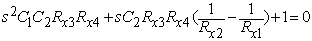(2)

From equation (2), the condition of oscillation (CO) and frequency of oscillation (FO) can be expressed as:

CO: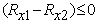and FO: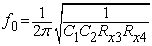(3)

Thus, from equation (3), it is clear that the resistors which are in CO, do not appear in FO, hence both CO and FO are fully uncoupled. Since Rx is tunable by bias current (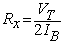), both CO and FO are independently electronically controllable. Independent controllability of FO is useful in realization of current controlled oscillators while control of CO independently is advantageous to incorporate amplitude stabilization.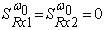(4)

From equation (4) it is seen that the proposed oscillator circuits enjoy low sensitivities.

3. Frequency Stability Analysis

Frequency stability is an important figure of merit on the basis of which different sinusoidal oscillators can be compared. We use the definition of frequency stability factor to be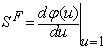, where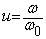is the normalized frequency and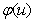represents the phase of the open loop transfer function of the oscillator circuits. The open loop transfer function (with link broken at point ‘P’ as shown in Fig. 2) is given by: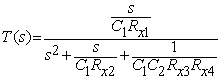(5)

Thus, from equation (5), the phase of the T(s) with the selection of C1 = C2 = C, Rx1 = Rx2 = Rx3 = Rx and Rx4 = Rx/n, is represented by the following equation: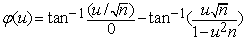(6)

Now differentiating equation (6) with respect to u, we obtain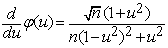, hence, the value of SF for both the oscillators is obtained to be 2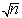. Thus, the new current controlled oscillators offer very good frequency stability factor for larger values of n. Similarly, if we vary both the resistors Rx3 and Rx4 simultaneously i.e., Rx3 = Rx4 = R/n,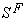will become 2n. Therefore, excellent frequency stability can be obtained by selecting larger values of n.

4. Simulation Results

To evaluate the workability of the proposed new current controlled oscillator circuits, SPICE simulations have been performed using the bipolar transistor parameters of PR100N (PNP) and NR100N (NPN) . The capacitor values used were C1 = C2 = 100nF, the condition of oscillation was established through the variation of Rx2 as a function of the D.C. bias current IB2 while keeping Rx1 fixed. The CCCIIs were biased with ± 2.5V D.C. power supplies. The value of Rx3 was fixed through IB3 and the control of frequency was done through the variation of IB4.Download asVeiw figureFigures index
Figure 3(a). PSPICE simulation results (Transient waveform)Download asVeiw figureFigures index
Figure 3(b). PSPICE simulation results (Steady state response)Download asVeiw figureFigures index
Figure 3(c). Spectrum of the output waveformDownload asVeiw figureFigures index
Figure 3(d). Results of the Monte-Carlo Analysis

The SPICE generated transient and steady state responses of the oscillator output voltage are shown in Figure 3(a) and Figure 3(b) respectively. Figure 3(c) shows the frequency spectrum which clearly shows the THD as 2.4% only which is reflective of good spectral purity of the generated signal. To check the influence of random variations in the parameter and element values on the startup of the oscillations, a Monte Carlo simulation was carried out whose results are shown in Figure 3(d). The results in Figure 3(d) demonstrate that component/parameter tolerances do not have much effect on the build-up of the oscillations of the proposed oscillator.

The simulation results of Figure 3(a)-(d), thus, confirm the workability of the proposed oscillator configuration.

5. Concluding Remarks

Although a number of current-controlled sinusoidal oscillators employing the controlled current conveyors as an active element [1-12] have been proposed in the earlier literature, from a review of the earlier works it was found that any sinusoidal structure providing fully uncoupled tuning laws for oscillation condition as well as frequency of oscillation had not been reported earlier. This paper introduced a circuit of this kind using four CCCIIs and two grounded capacitors. The proposed circuit has excellent frequency stability. The workability of proposed circuit was demonstrated by PSPICE simulations.

Acknowledgement

The authors wish to thank an anonymous reviewer for his constructive comments and suggestions.

References

  Fabre, A., Saaid, O., Wiest F.and Boucheron C., “Current controlled bandpass filter based on translinear conveyors”, Electronics Letters, 31(29), 1727-1728, September 1995.In article  Fabre, A., Saaid, O., Wiest F.and BoucheronC., “High frequency applications based on a new current controlled conveyor”, IEEE Trans Circuits Syst, 43(2), 82-91, February1996.In article View Article  Kiranon, W., Kesorn, J. and Wardkein, P., “Current controlled oscillator based on translinear conveyors”, Electronics Letters, 32(15), 1330-1331, July 1996.In article View Article  Kiranon, W., Kesorn, J., Sangpisit, W. and Kamprasert, N.,“Electronically tunable multifunctional translinear-C filter and oscillator”, Electronics Letters, 33(7), 573-574, March 1997.In article View Article  Abuelma’atti M.T. and Tasadduq, N. A., “A novel current controlled oscillator using translinear current conveyors”, Frequenz, 52(5-6), 123-124, 1998.In article  Abuelma’atti M.T. and Al-Qahtani, M.A. “A new current controlled multiphase sinusoidal oscillator using two translinear conveyors”, IEEE Trans Circuits Syst–II, 45(7), 881-885, July1998.In article View Article  Horng, J.W., “A sinusoidal oscillator using current-controlled current conveyors”, Int. J. Electronics, 88(6), 659-664, 2001.In article View Article  Fongsamut,C., Anuntahirunrat, K., Kumwachara K.nd Surakampontorn, W.,“Current-conveyor-based single-element-controlled and current-controlled sinusoidal oscillators”, Int. J. Electronics, 93(7), 467-478, July 2006.In article View Article  Maheshwari, S., “Current-mode third-order quadrature oscillator”, IET Circuits Devices Syst, 4(3), 188-195, 2010.In article View Article  Bhaskar D. R. and Senani, R., “New CFOA-based single-element-controlled sinusoidal oscillators”, IEEE Trans Instrumentation and Measurement, 55(6), 2014-2021, December 2006.In article View Article  Senani, R., Bhaskar D. R. and Singh, A. K., “Current Conveyors: variants, applications and hardware implementations”, Springer International Publishing, Switzerland, 2015, Ch. 8, pp. 395-423.In article View Article  Senani, R., Bhaskar D. R. and Singh, A. K., “Sinusoidal Oscillators and Waveform generators using modern electronic circuit building blocks”, Springer International Publishing, Switzerland, Ch. 8, pp. 395-425, 2016.In article View Article  Frey, D.R., “Log domain filtering: an approach to current-mode filtering”, IEE Proc. G., Circuits Devices Syst., 140(6), 406-416, December 1993.In article View Article